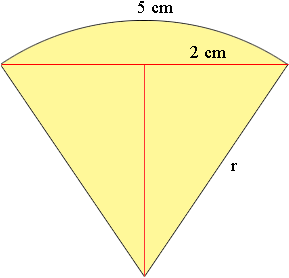SEARCH HOMEMath Central Quandaries & QueriesQuestion from Larissa, a student: In a circle, the length of a chord AB is 4 cm and the length of the arc AB is 5 cm. Find the central angle theta, in radians, correct to four decimal places. Then give the answer to the nearest degree. I think I'm supposed to use Newton's method, but am not sure how to start with this problem.Hi Larissa,If the radius of the circle is r cm then you know that the length of the arc a is given by

a = r θ

Also from the diagram

sin(θ/2) = 2/r

Solve this equation for r and substitute into the first equation with a = 5. Now you can apply Newton's Method.

If you need more assistance write back,
HarleyMath Central is supported by the University of Regina and The Pacific Institute for the Mathematical Sciences.